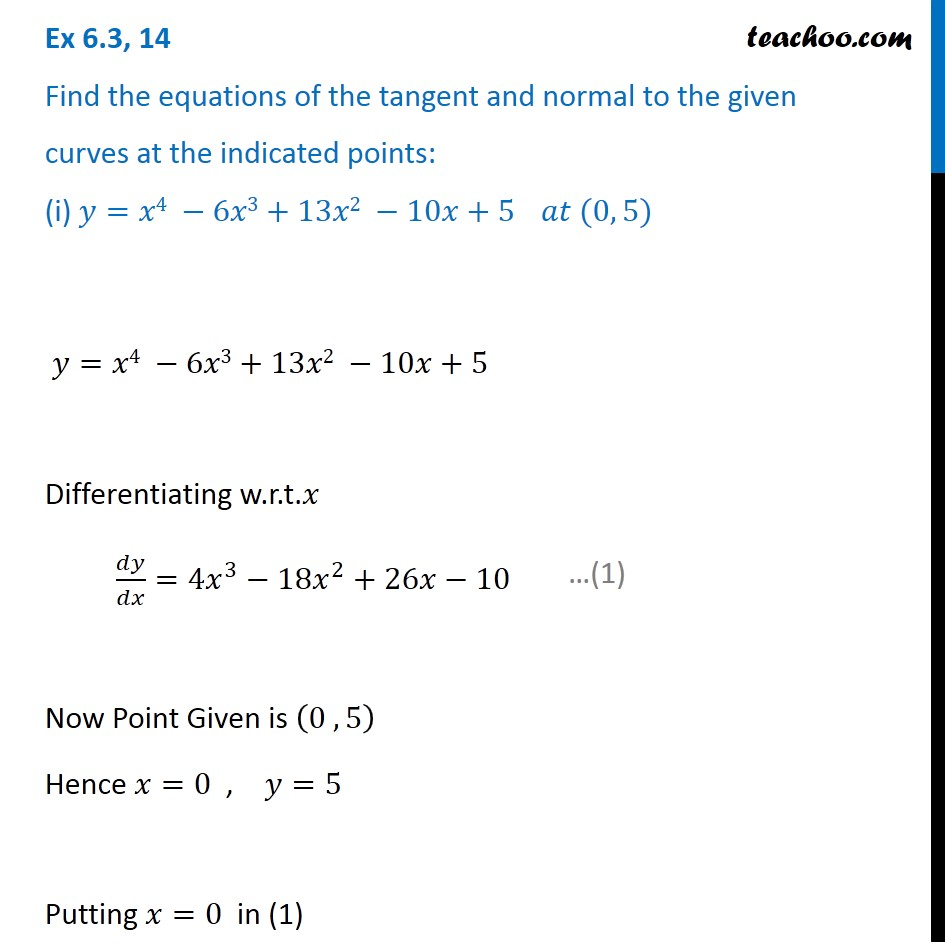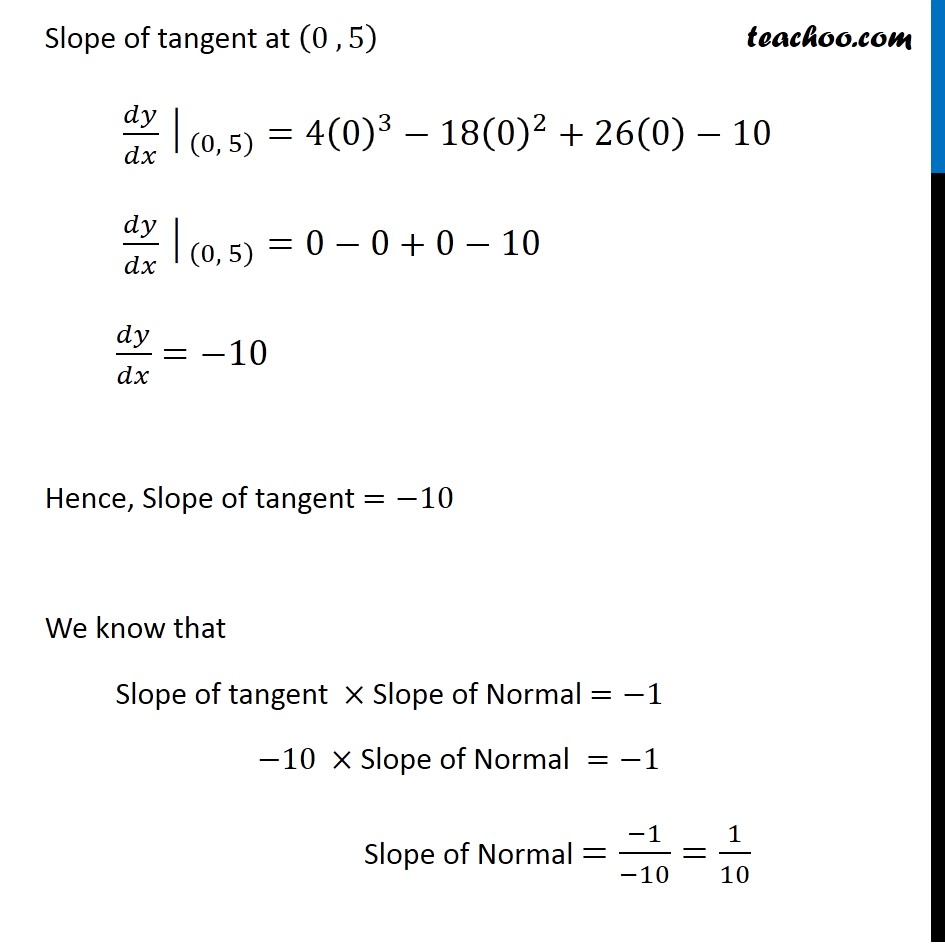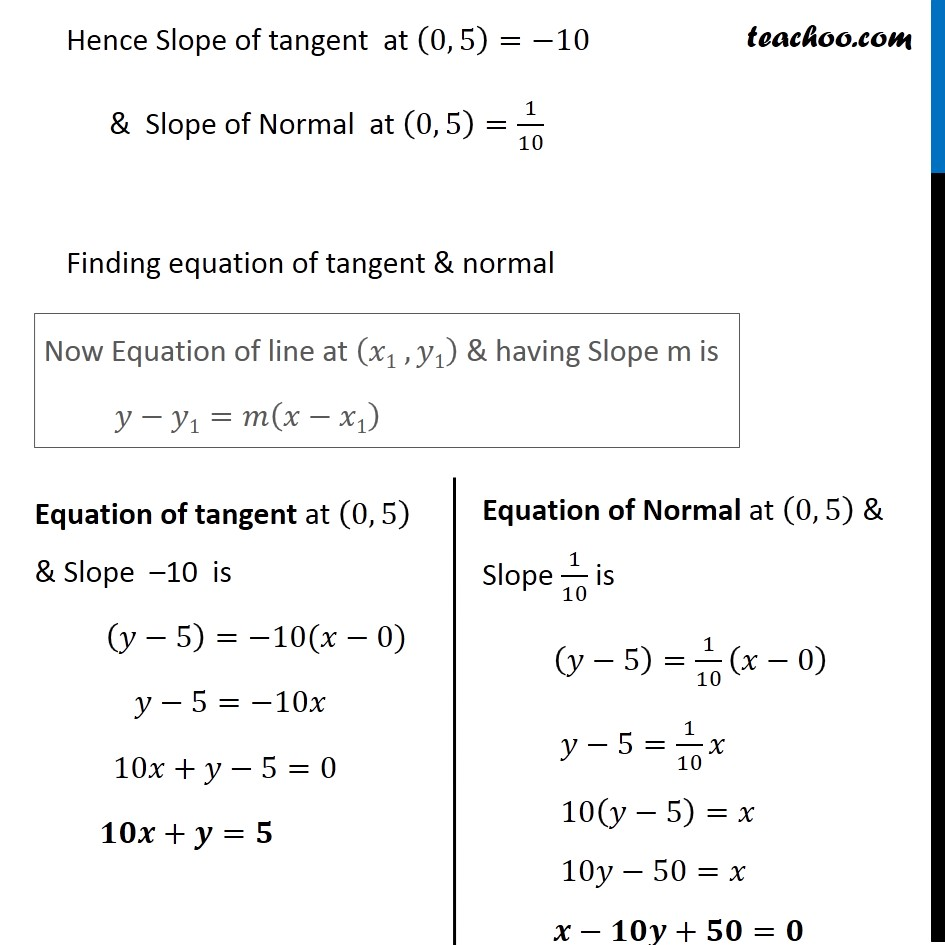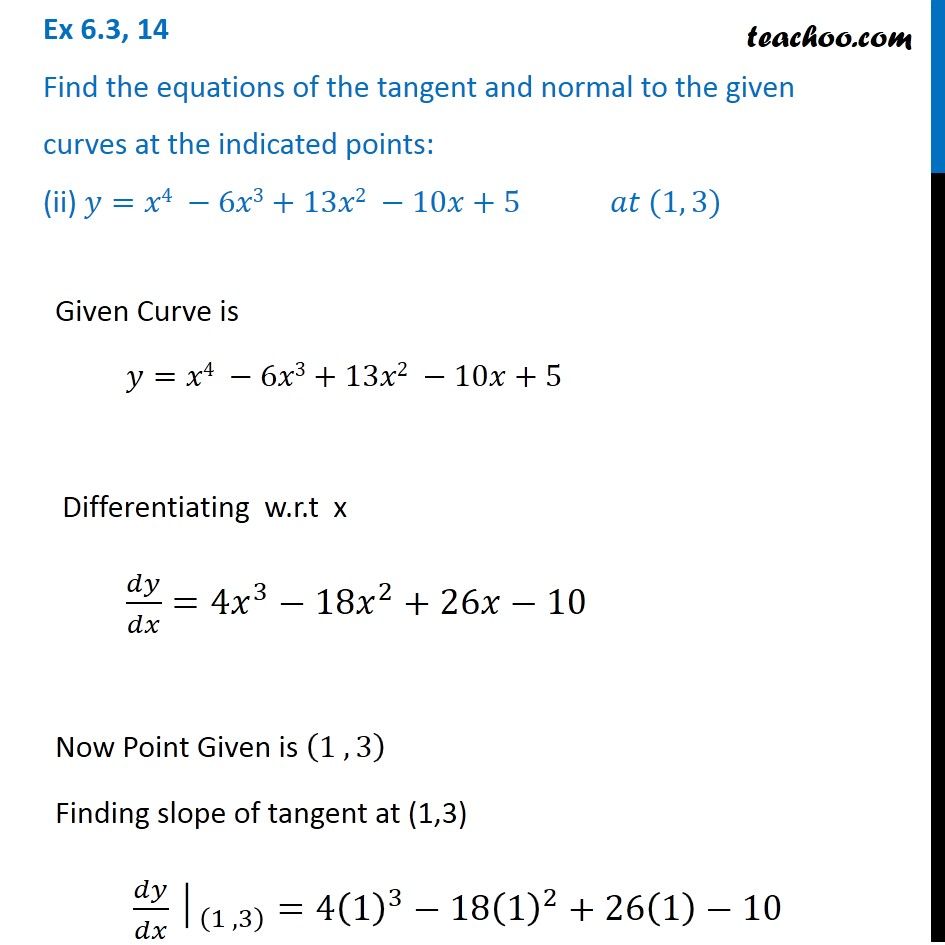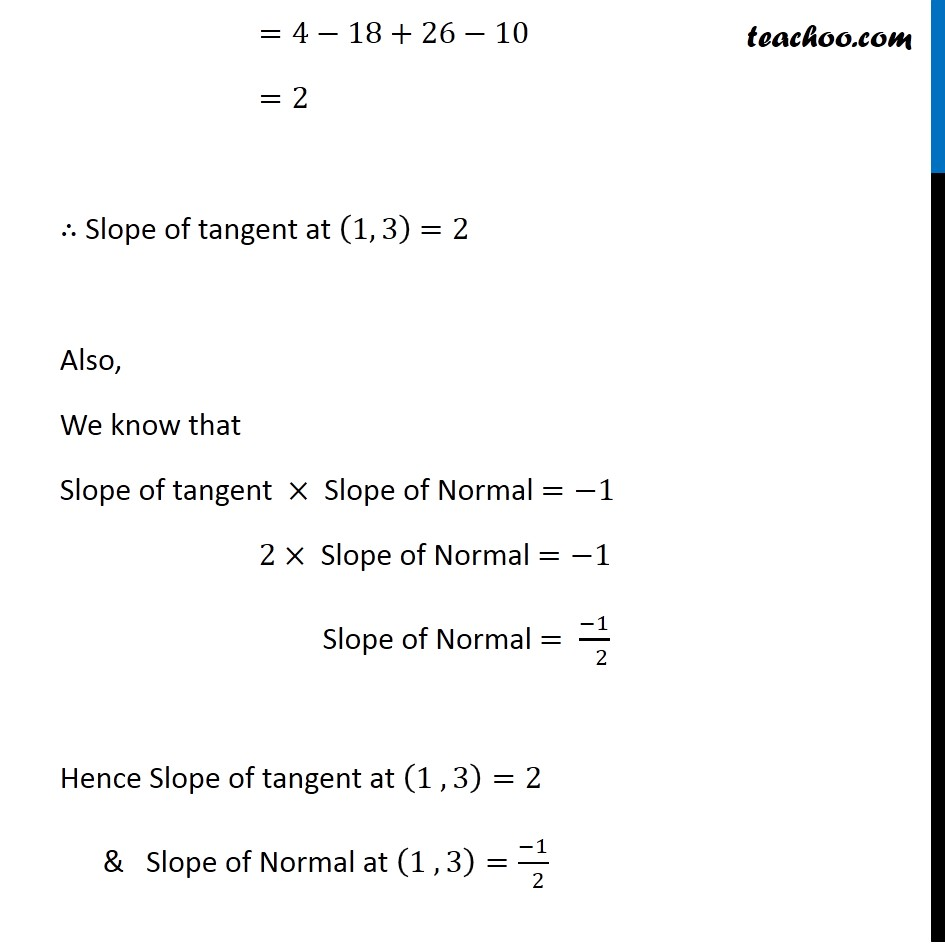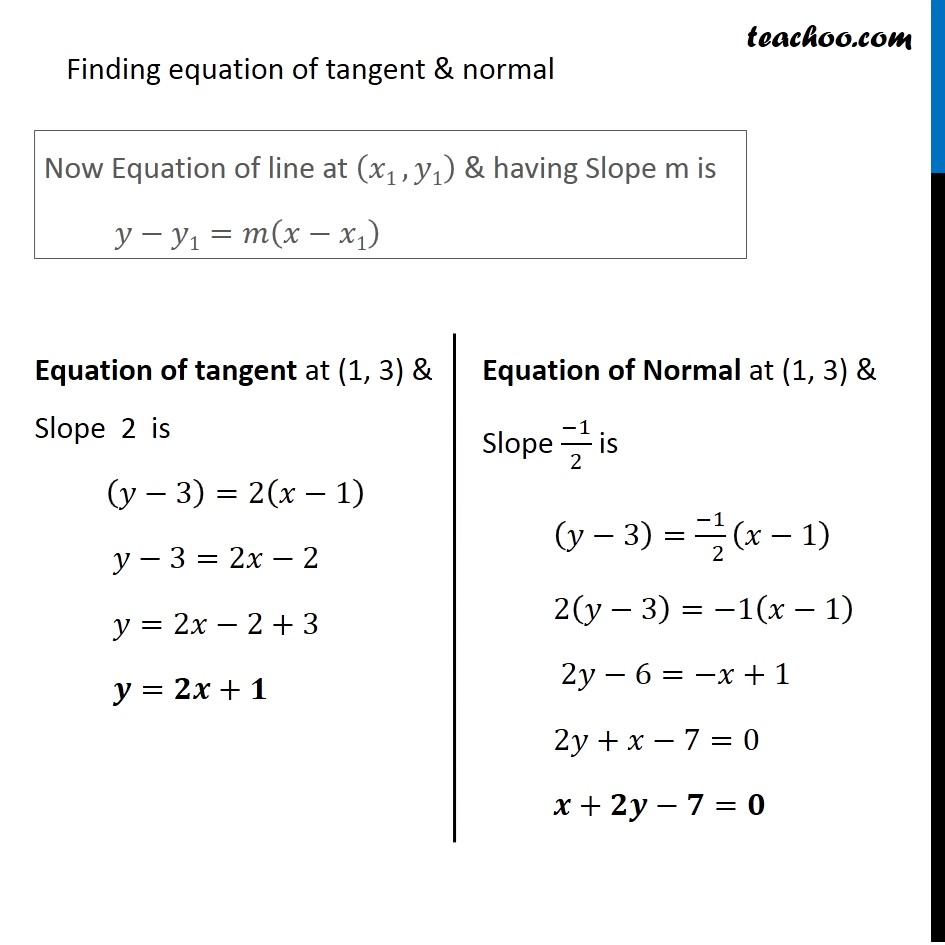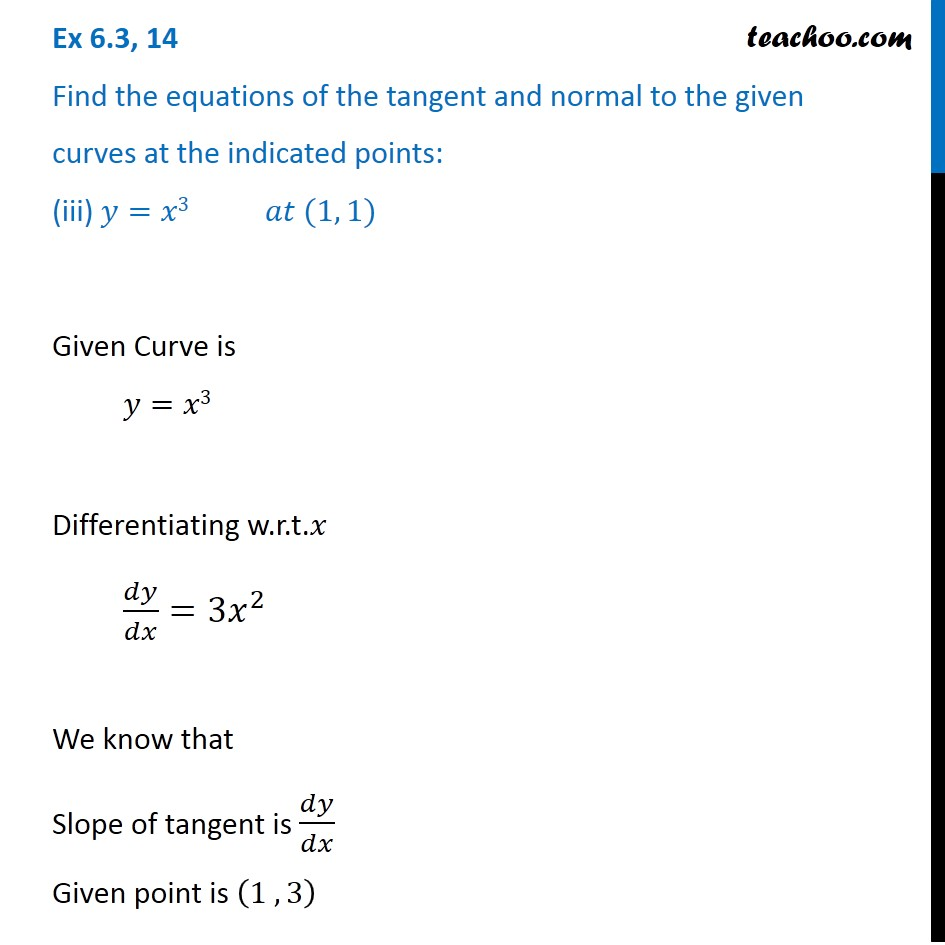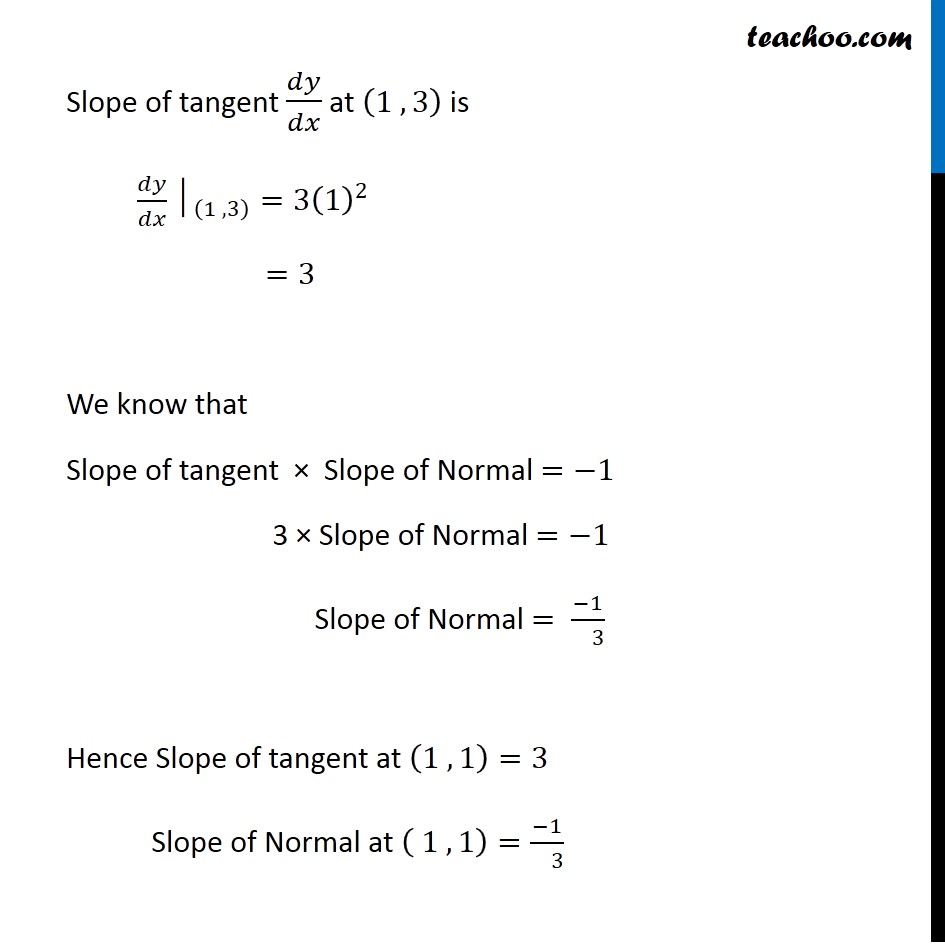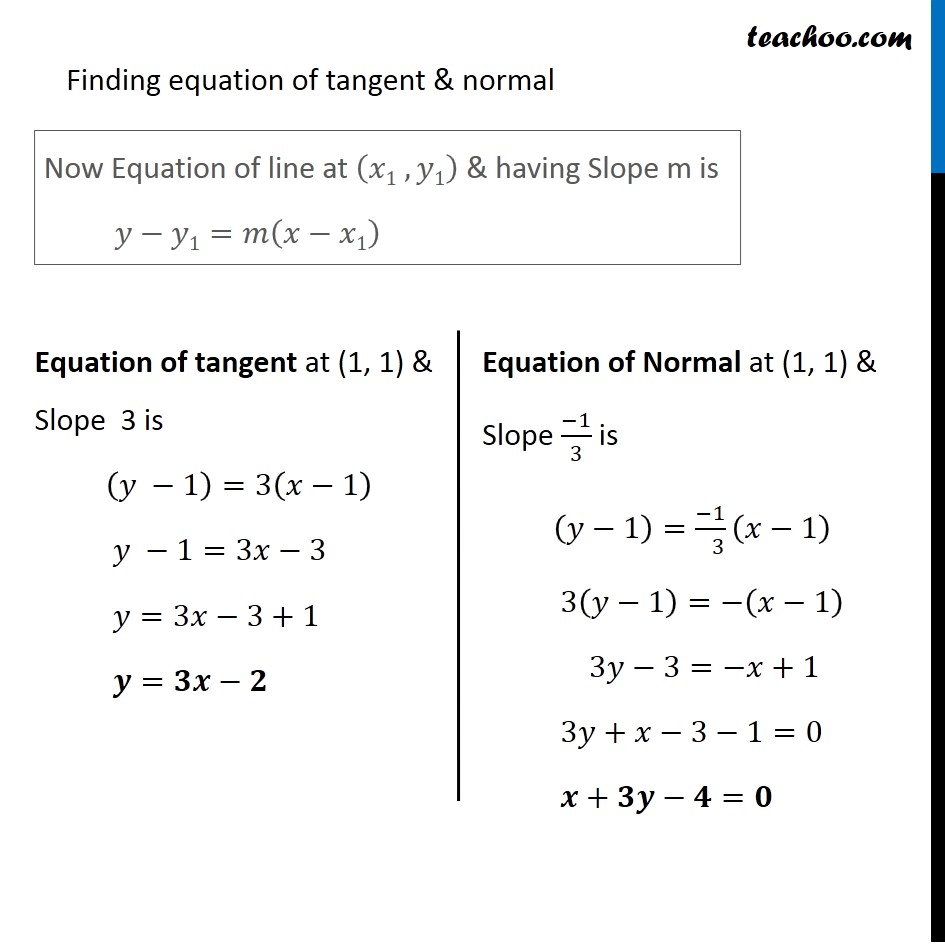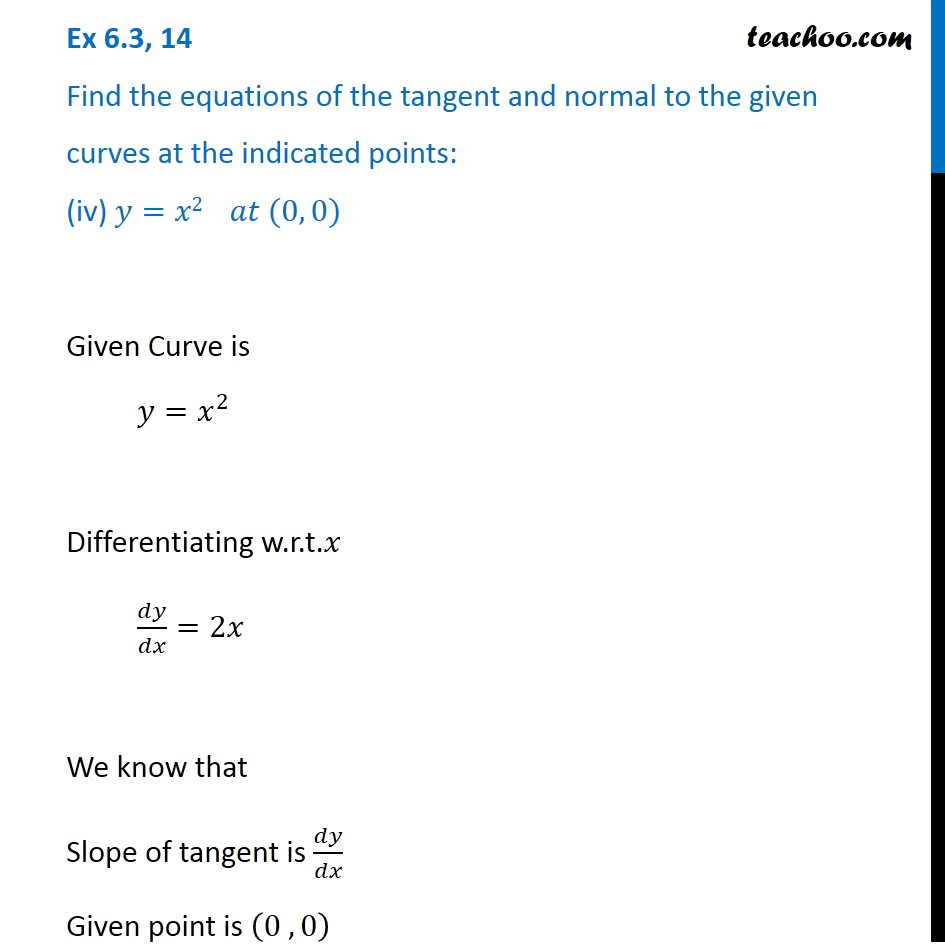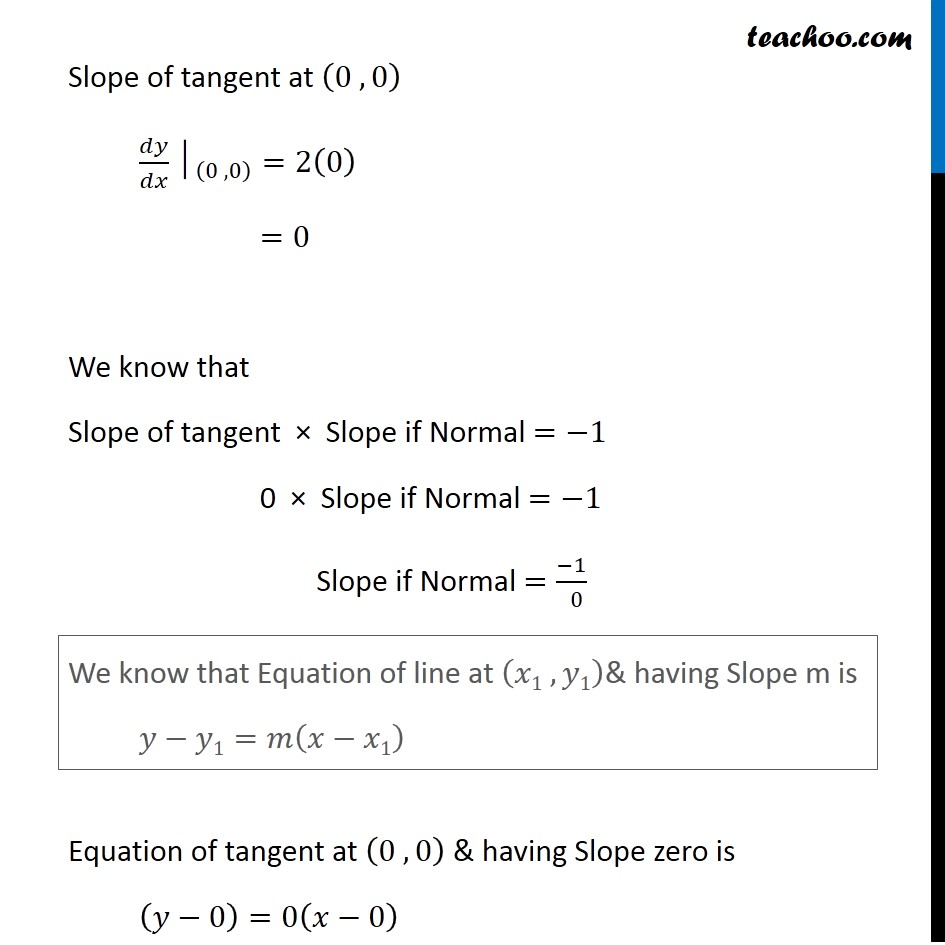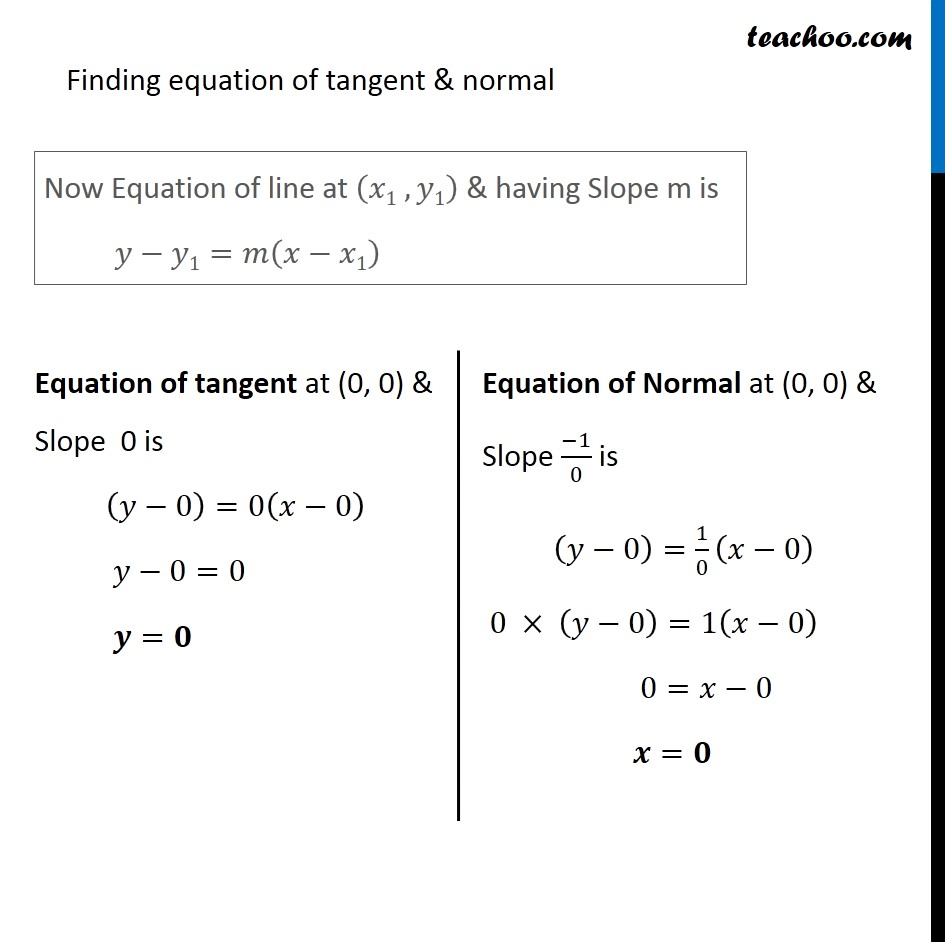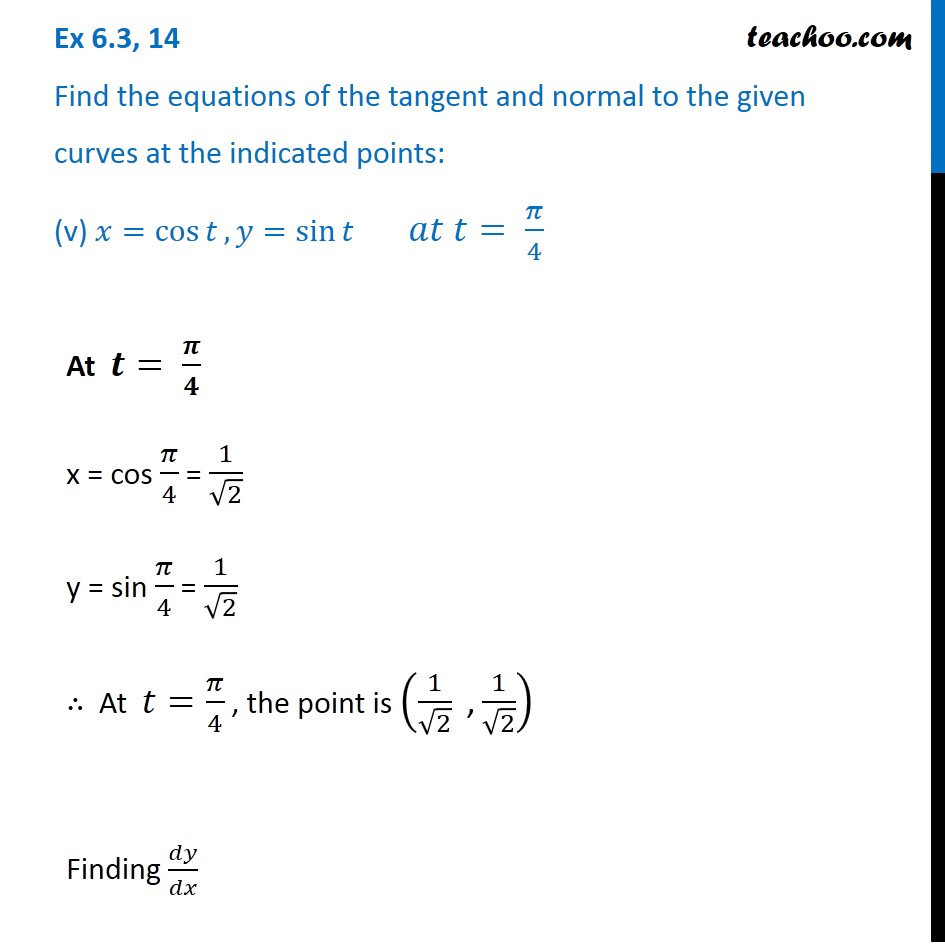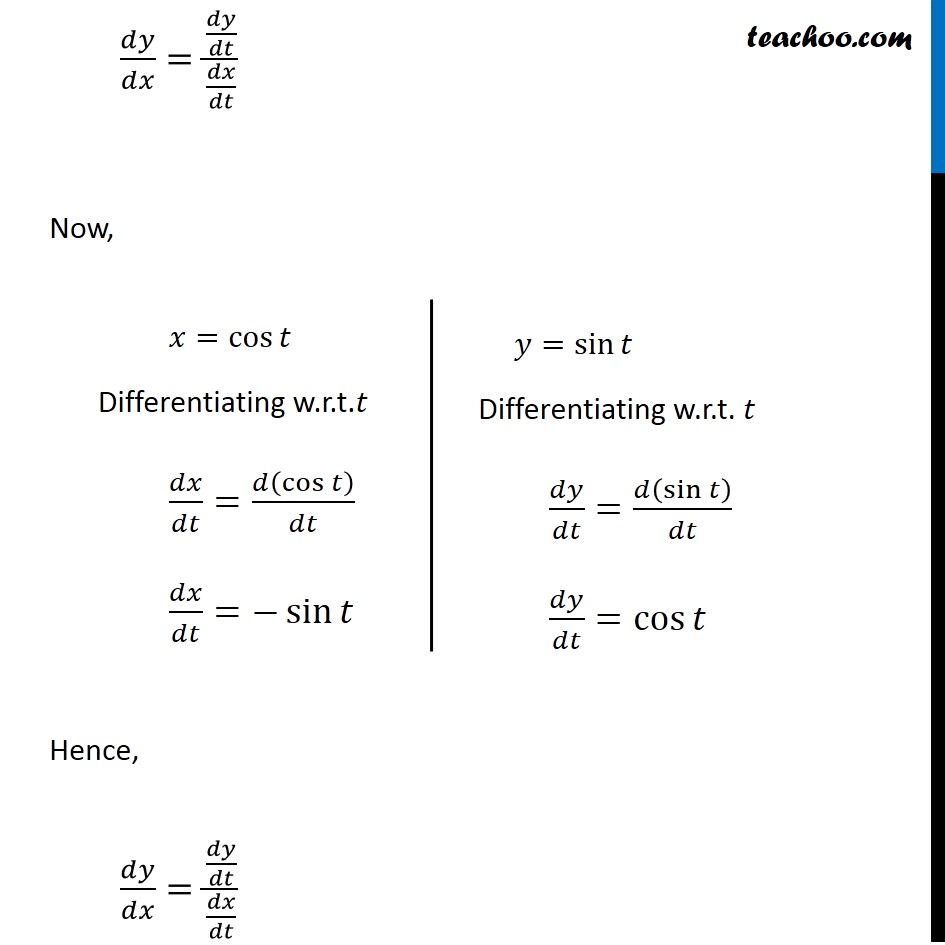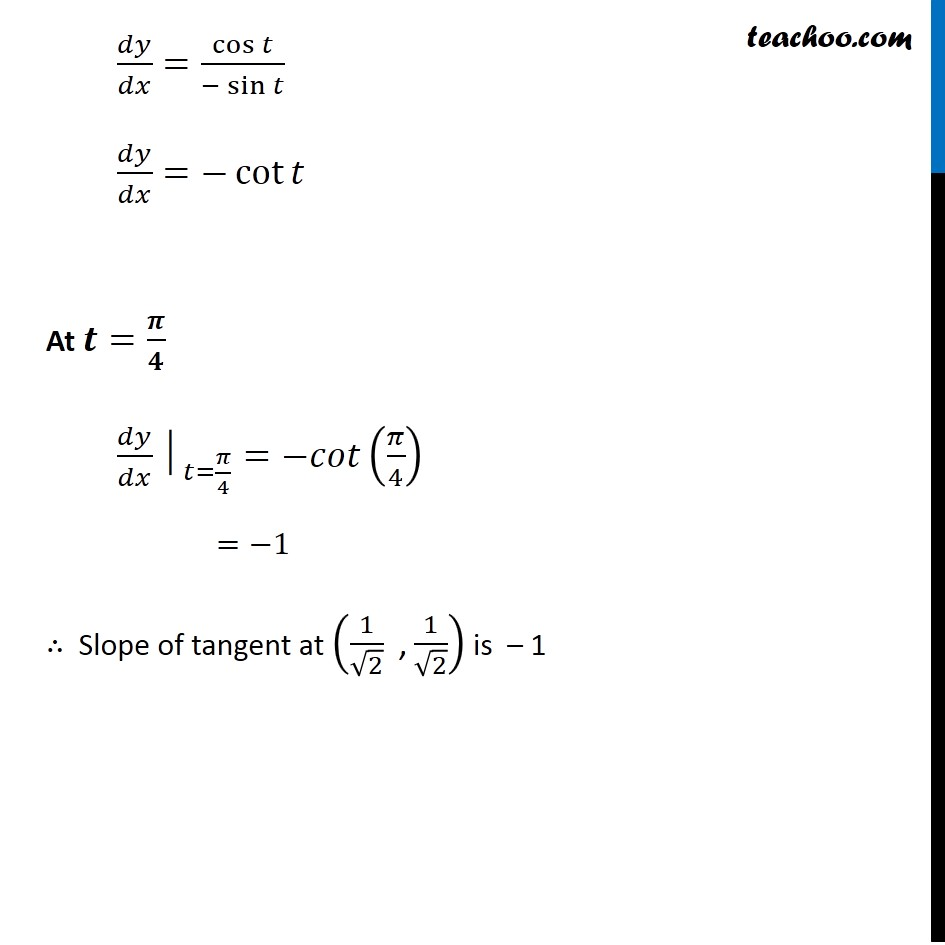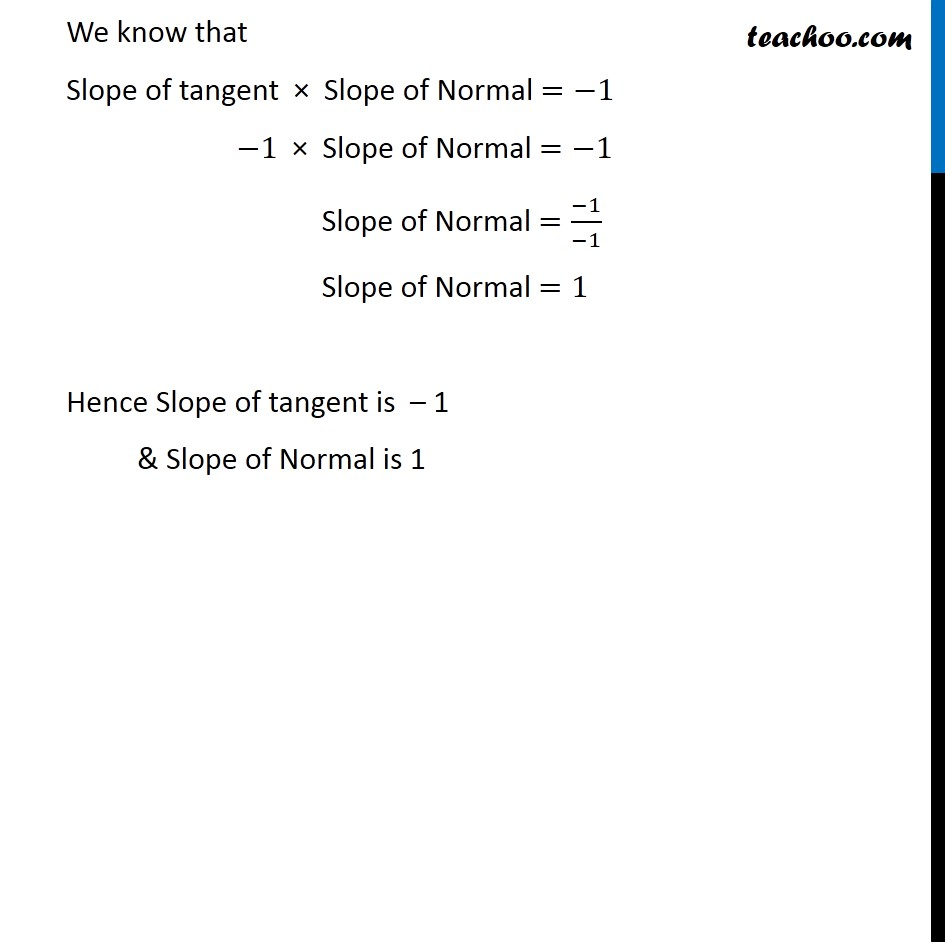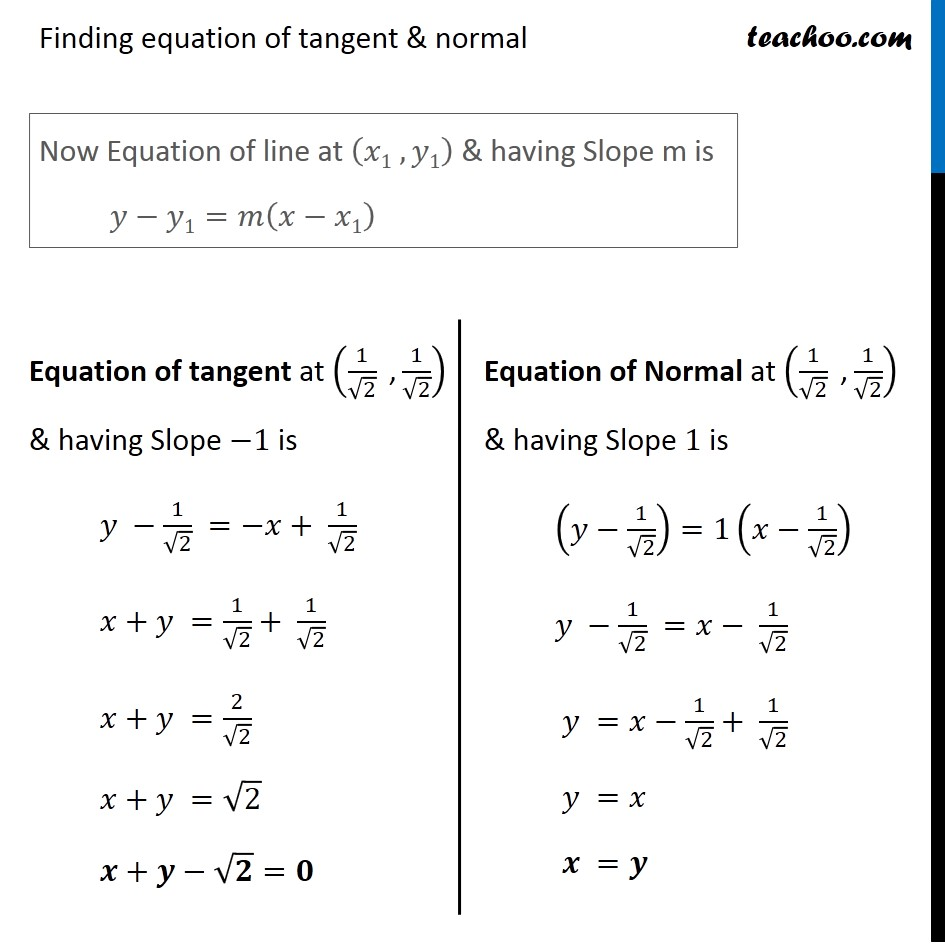1. Chapter 6 Class 12 Application of Derivatives
2. Concept wise
3. Finding equation of tangent/normal when point and curve is given

Transcript

Ex 6.3, 14 Find the equations of the tangent and normal to the given curves at the indicated points: (i) 𝑦=𝑥4 −6𝑥3+13𝑥2 −10𝑥+5 𝑎𝑡 (0, 5) 𝑦=𝑥4 −6𝑥3+13𝑥2 −10𝑥+5 Differentiating w.r.t. 𝑥 𝑑𝑦/𝑑𝑥=4𝑥^3−18𝑥^2+26𝑥−10 Now Point Given is (0 ,5) Hence 𝑥=0 , 𝑦=5 Putting 𝑥=0 in (1) Slope of tangent at (0 , 5) 〖𝑑𝑦/𝑑𝑥│〗_((0, 5) )=4(0)^3−18(0)^2+26(0)−10 〖𝑑𝑦/𝑑𝑥│〗_((0, 5) )=0−0+0−10 𝑑𝑦/𝑑𝑥=−10 Hence, Slope of tangent =−10 We know that Slope of tangent × Slope of Normal =−1 −10 ×"Slope of Normal "=−1 "Slope of Normal" =(−1)/(−10)=1/10 Hence Slope of tangent at (0, 5)=−10 & Slope of Normal at (0, 5)=1/10 Finding equation of tangent & normal Now Equation of line at (𝑥1 , 𝑦1) & having Slope m is 𝑦−𝑦1=𝑚(𝑥−𝑥1) Equation of tangent at (0, 5) & Slope –10 is (𝑦−5)=−10(𝑥−0) 𝑦−5=−10𝑥 10𝑥+𝑦−5=0 𝟏𝟎𝒙+𝒚=𝟓 Equation of Normal at (0, 5) & Slope 1/10 is (𝑦−5)=1/10 (𝑥−0) 𝑦−5=1/10 𝑥 10(𝑦−5)=𝑥 10𝑦−50=𝑥 𝒙−𝟏𝟎𝒚+𝟓𝟎=𝟎 Ex 6.3, 14 Find the equations of the tangent and normal to the given curves at the indicated points: (ii) 𝑦=𝑥4 −6𝑥3+13𝑥2 −10𝑥+5 𝑎𝑡 (1, 3) Given Curve is 𝑦=𝑥4 −6𝑥3+13𝑥2 −10𝑥+5 Differentiating w.r.t x 𝑑𝑦/𝑑𝑥=4𝑥^3−18𝑥^2+26𝑥−10 Now Point Given is (1 , 3) Finding slope of tangent at (1,3) 〖𝑑𝑦/𝑑𝑥│〗_((1 , 3) )=4(1)^3−18(1)^2+26(1)−10 =4−18+26−10 =2 ∴ Slope of tangent at (1, 3) =2 Also, We know that Slope of tangent × Slope of Normal =−1 2× Slope of Normal =−1 Slope of Normal = (−1)/( 2) Hence Slope of tangent at (1 , 3)=2 & Slope of Normal at (1 , 3)=(−1)/( 2) Finding equation of tangent & normal Now Equation of line at (𝑥1 , 𝑦1) & having Slope m is 𝑦−𝑦1=𝑚(𝑥−𝑥1) Equation of tangent at (1, 3) & Slope 2 is (𝑦−3)=2(𝑥−1) 𝑦−3=2𝑥−2 𝑦=2𝑥−2+3 𝒚=𝟐𝒙+𝟏 Equation of Normal at (1, 3) & Slope (−1)/2 is (𝑦−3)=(−1)/( 2) (𝑥−1) 2(𝑦−3)=−1(𝑥−1) 2𝑦−6=−𝑥+1 2𝑦+𝑥−7=0 𝒙+𝟐𝒚−𝟕=𝟎 Ex 6.3, 14 Find the equations of the tangent and normal to the given curves at the indicated points: (iii) 𝑦=𝑥3 𝑎𝑡 (1, 1) Given Curve is 𝑦=𝑥3 Differentiating w.r.t.𝑥 𝑑𝑦/𝑑𝑥=3𝑥^2 We know that Slope of tangent is 𝑑𝑦/𝑑𝑥 Given point is (1 , 3) Slope of tangent 𝑑𝑦/𝑑𝑥 at (1 , 3) is 〖𝑑𝑦/𝑑𝑥│〗_((1 , 3) )=3(1)^2 =3 We know that Slope of tangent × Slope of Normal =−1 3 × Slope of Normal =−1 Slope of Normal = (−1)/( 3) Hence Slope of tangent at (1 , 1)=3 Slope of Normal at ( 1 , 1)=(−1)/( 3) Finding equation of tangent & normal Now Equation of line at (𝑥1 , 𝑦1) & having Slope m is 𝑦−𝑦1=𝑚(𝑥−𝑥1) Equation of tangent at (1, 1) & Slope 3 is (𝑦 −1)=3(𝑥−1) 𝑦 −1=3𝑥−3 𝑦=3𝑥−3+1 𝒚=𝟑𝒙−𝟐 Equation of Normal at (1, 1) & Slope (−1)/3 is (𝑦−1)=(−1)/( 3) (𝑥−1) 3(𝑦−1)=−(𝑥−1) 3𝑦−3=−𝑥+1 3𝑦+𝑥−3−1=0 𝒙+𝟑𝒚−𝟒=𝟎 Ex 6.3, 14 Find the equations of the tangent and normal to the given curves at the indicated points: (iv) 𝑦=𝑥2 𝑎𝑡 (0, 0) Given Curve is 𝑦=𝑥^2 Differentiating w.r.t.𝑥 𝑑𝑦/𝑑𝑥=2𝑥 We know that Slope of tangent is 𝑑𝑦/𝑑𝑥 Given point is (0 , 0) Slope of tangent at (0 , 0) 〖𝑑𝑦/𝑑𝑥│〗_((0 , 0) )=2(0) =0 We know that Slope of tangent × Slope if Normal =−1 0 × Slope if Normal =−1 Slope if Normal =(−1)/( 0) Equation of tangent at (0 , 0) & having Slope zero is (𝑦−0)=0(𝑥−0) We know that Equation of line at (𝑥1 , 𝑦1)& having Slope m is 𝑦−𝑦1=𝑚(𝑥−𝑥1) Finding equation of tangent & normal Now Equation of line at (𝑥1 , 𝑦1) & having Slope m is 𝑦−𝑦1=𝑚(𝑥−𝑥1) Equation of tangent at (0, 0) & Slope 0 is (𝑦−0)=0(𝑥−0) 𝑦−0=0 𝒚=𝟎 Equation of Normal at (0, 0) & Slope (−1)/0 is (𝑦−0)=1/0 (𝑥−0) 0 × (𝑦−0)=1(𝑥−0) 0=𝑥−0 𝒙=𝟎 Ex 6.3, 14 Find the equations of the tangent and normal to the given curves at the indicated points: (v) 𝑥=cos⁡𝑡, 𝑦=sin⁡𝑡 𝑎𝑡 𝑡= 𝜋/4 At 𝒕= 𝝅/𝟒 x = cos 𝜋/4 = 1/√2 y = sin 𝜋/4 = 1/√2 ∴ At 𝑡=𝜋/4 , the point is (1/√2 " ," 1/√2) Finding 𝑑𝑦/𝑑𝑥 𝑑𝑦/𝑑𝑥=(𝑑𝑦/𝑑𝑡)/(𝑑𝑥/𝑑𝑡) Now, Hence, 𝑑𝑦/𝑑𝑥=(𝑑𝑦/𝑑𝑡)/(𝑑𝑥/𝑑𝑡) 𝑥=cos⁡𝑡 Differentiating w.r.t.𝑡 𝑑𝑥/𝑑𝑡=𝑑(cos⁡𝑡 )/𝑑𝑡 𝑑𝑥/𝑑𝑡=−sin⁡𝑡 𝑦=sin⁡𝑡 Differentiating w.r.t. 𝑡 𝑑𝑦/𝑑𝑡=𝑑(sin⁡𝑡 )/𝑑𝑡 𝑑𝑦/𝑑𝑡=cos⁡𝑡 𝑑𝑦/𝑑𝑥=cos⁡𝑡/(−sin⁡𝑡 ) 𝑑𝑦/𝑑𝑥=−cot⁡𝑡 At 𝒕=𝝅/𝟒 〖𝑑𝑦/𝑑𝑥│〗_(𝑡=𝜋/4)=−𝑐𝑜𝑡(𝜋/4) =−1 ∴ Slope of tangent at (1/√2 " ," 1/√2) is – 1 We know that Slope of tangent × Slope of Normal =−1 −1 × Slope of Normal =−1 Slope of Normal =(−1)/(−1) Slope of Normal =1 Hence Slope of tangent is – 1 & Slope of Normal is 1 Finding equation of tangent & normal Now Equation of line at (𝑥1 , 𝑦1) & having Slope m is 𝑦−𝑦1=𝑚(𝑥−𝑥1) Equation of tangent at (1/√2 " ," 1/√2) & having Slope −1 is 𝑦 −1/√2 =−𝑥+ 1/√2 𝑥+𝑦 =1/√2+ 1/√2 𝑥+𝑦 =2/√2 𝑥+𝑦 =√2 𝒙+𝒚−√𝟐=𝟎 Equation of Normal at (1/√2 " ," 1/√2) & having Slope 1 is (𝑦−1/√2)=1(𝑥−1/√2) 𝑦 −1/√2 =𝑥− 1/√2 𝑦 =𝑥−1/√2+ 1/√2 𝑦 =𝑥 𝒙 =𝒚

Finding equation of tangent/normal when point and curve is given